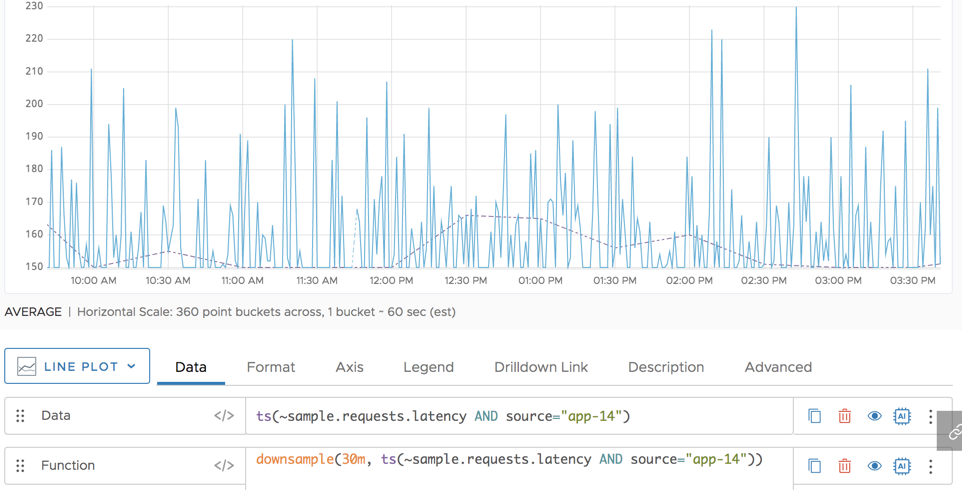Reference to the downsample() function

## Summary

``````downsample(<timeWindow>, <expression>)
``````

Returns the values in the expression that occur in each time window. For example, `downsample(30m, ts(my.metric)` returns the values of `my.metric` every half hour.

## Parameters

ParameterDescription
timeWindow The filter time window. The function filters the data so you see only the data for the specified time window.
expression Expression to downsample.

## Description

The `downsample()` function allows you to filter any `ts()` expression to just the values occurring every `timeWindow`.

For example, to see the values for every half-hour of a given metric, enter the following `ts()` expression:

``` downsample(30, ts(“my.metric”)) ```

## Examples

The following example shows the CPU load average for a specified source (blue line). The dashed orange line shows only one value every 30 minutes.Series Matching